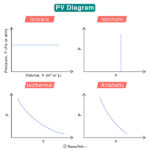Home / Physics / Blackbody Radiation

A blackbody is a hypothetical object that absorbs all radiation falling on it and reflects and transmits none. A blackbody is also a perfect emitter of radiation. It emits radiation across all wavelengths – the phenomenon known as blackbody radiation. The thermal energy spectrum of a blackbody shows the radiation intensity over a range of wavelengths or frequencies. However, there is one wavelength or frequency where the radiation has the highest intensity.

The attributes of blackbody radiation can be described in terms of several laws governed by quantum theory.

### 1. Planck’s Law

Planck’s law gives the amount of radiation emitted per unit solid angle in terms of wavelength or frequency and equilibrium temperature. According to Planck’s law, the spectral radiance at a given temperature is given by

$B(\lambda ,T) = \frac{2hc^2}{\lambda^5}\frac{1}{e^{\frac{hc}{\lambda k_B T}} – 1}$

Where

T: Absolute temperature

c: Speed of light

### 2. Wien’s Law or Wien’s Displacement Law

Wien’s law states that the wavelength at which a blackbody emits radiation with maximum intensity is inversely proportional to the absolute temperature.

$\lambda_{max} = \frac{b}{T}$

Where

λmax: Wavelength at which the radiation intensity is maximum, known as peak wavelength

b: A constant called Wien’s constant, whose value is 2.897 x 10-3 m·K

T: Absolute temperature

### 3. Stefan-Boltzmann’s Law

Stefan-Boltzmann’s law states that the total radiant power emitted by a surface across all wavelengths is proportional to the fourth power of its absolute temperature. Therefore, the total energy radiated by a blackbody depends on its temperature.

$\frac{P}{A} = \sigma T^4$

A: Surface area

T: Absolute temperature

The blackbody radiation graphs at different temperatures are shown below.

These curves have the following features:

1. The shape of the curve is continuous and complex, as described by Planck’s law.

2. Each curve passes through a maximum corresponding to its peak wavelength.

3. The peak wavelength decreases with temperature, as stated by Wien’s law.

4. The intensity of radiation increases as the temperature increases.

5. The total radiated energy (the area under the curves) increases rapidly as the temperature increases, a consequence of Stefan-Boltzmann’s law.

6. All blackbodies above absolute zero temperature emit radiation, although the intensities are low at short and long wavelengths.Brownian MotionPV DiagramVelocity vs AccelerationAcceleration due to Gravity